• 散点图
2021-06-07 10:00:09

接续博文 “【Python_绘图】折线图” CSDN

# 功能

• 数据：读取表格（可指定Sheet表……也可指定Sheet表中的特定列）
• 绘图样式：多条折线图+散点图 → 标记点折线图
• 设置参数：设置轴标题、添加分界线等

# 代码

import matplotlib.pyplot as plt
import xlrd
import numpy as np
import seaborn as sns

# 设置字体格式，以显示中文
plt.rcParams['font.sans-serif'] = ['Times New Roman']

x_data = []
y1_data = []
y2_data = []
y3_data = []
y4_data = []
y5_data = []
y6_data = []

# 读取表格数据
data = xlrd.open_workbook(r'D:\mekong_NLP\作图\20210512 作图数据汇总\图三 情感折线与词云\20210606 情感分值作图表.xlsx')
table = data.sheets()  # 表格中的Sheet1
x_data = list(range(1971,2021,1))
x_ticks=np.arange(1971,2021,5)

#读取第x行
cap1 = table.row_values(0)
cap2 = table.row_values(1)
cap3 = table.row_values(2)
cap4 = table.row_values(3)
cap5 = table.row_values(4)
cap6 = table.row_values(5)

for i in range(0, len(x_data)):
y1_data.append(cap1[i])
for i in range(0, len(x_data)):
y2_data.append(cap2[i])
for i in range(0, len(x_data)):
y3_data.append(cap3[i])
for i in range(0, len(x_data)):
y4_data.append(cap4[i])
for i in range(0, len(x_data)):
y5_data.append(cap5[i])
for i in range(0, len(x_data)):
y6_data.append(cap6[i])

#逐条 作折线图
plt.plot(x_data, y1_data, c='crimson',linestyle=':',linewidth=2,label='China')
plt.plot(x_data, y2_data, c='coral',linestyle=':',linewidth=2,label='Myanmar')
plt.plot(x_data, y3_data, c='hotpink',linestyle=':',linewidth=2,label='Laos')
plt.plot(x_data, y4_data, c='blue',linestyle=':',linewidth=2,label='Thailand')
plt.plot(x_data, y5_data, c='lightsteelblue',linestyle=':',linewidth=2,label='Cambodia')
plt.plot(x_data, y6_data, c='cornflowerblue',linestyle=':',linewidth=2,label='Vietnam')
#逐条线 作散点图
plt.scatter(x_data, y1_data, c='crimson',s=15)
plt.scatter(x_data, y2_data, c='coral',s=15)
plt.scatter(x_data, y3_data, c='hotpink',s=15)
plt.scatter(x_data, y4_data, c='blue',s=15)
plt.scatter(x_data, y5_data, c='lightsteelblue',s=15)
plt.scatter(x_data, y6_data, c='cornflowerblue',s=15)

#修改图 相关参数
#plt.title('F1值随迭代次数的变化')
plt.xticks(x_ticks)
plt.tick_params(labelsize=16)#横纵标签大小
plt.xlabel('Year',size=16)
plt.ylabel('Sentiment score',size=16)
plt.ylim(ymin=-0.80, ymax=0.80)
plt.vlines(1991, -0.8, 0.8, colors="black", linestyles="dashed",linewidth=1)#分界线
plt.vlines(1994, -0.8, 0.8, colors="black", linestyles="dashed",linewidth=1)
plt.vlines(2008, -0.8, 0.8, colors="black", linestyles="dashed",linewidth=1)
plt.vlines(2015, -0.8, 0.8, colors="black", linestyles="dashed",linewidth=1)

leg=plt.legend(columnspacing=1,fontsize=16)#图例
leg.get_frame().set_linewidth(0.0)#不显示右边与上边线
sns.despine()#不显示右边与上边线
plt.show()#绘图

# 效果示例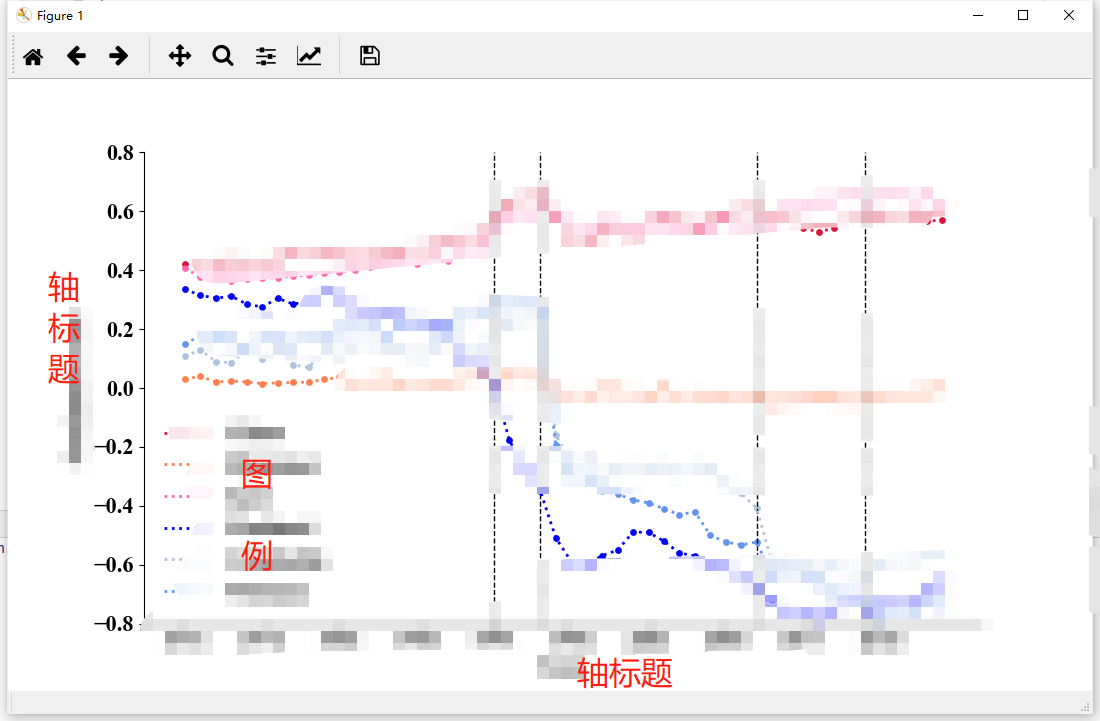# 附：使线贴合纵轴

plt.xlim(1971,2021)python
更多相关内容
• 主要为大家详细介绍了python2.7使用plotly绘制本地散点图折线图实例，具有一定的参考价值，感兴趣的小伙伴们可以参考一下
• 主要为大家详细介绍了python使用matplotlib模块绘制多条折线图散点图的方法，具有一定的参考价值，感兴趣的小伙伴们可以参考一下
• 这篇文章主要介绍了Python散点图折线图绘制过程解析,文中通过示例代码介绍的非常详细，对大家的学习或者工作具有一定的参考学习价值,需要的朋友可以参考下 在数据分析的过程中，经常需要将数据可视化，目前常使用...
• Qt5.8 使用自带的QChart实现改变折线图散点图X轴及图样式效果，这个积分是系统自己定的，不值这么多，有需要的可以私信（资源名+邮箱）
• 地图图表、柱状图、条形图、折线图、中国地图、世界地图、省市地图、仪表盘、雷达图、饼图、散点图、瀑布图、气泡图、堆叠图、热力图、桑基图、关系图、漏斗图、Axure原型、rp原型、产品原型、大屏设计必备组件 ...
• 商业级数据图表，它是一个纯JavaScript...折线图（区域图）、柱状图（条状图）、散点图（气泡图）、K线图、饼图（环形图） 雷达图（填充雷达图）、和弦图、力导向布局图、地图、仪表盘、漏斗图、事件河流图等12类图表JAR
• 散点图折线图是数据分析中最常用的两种图形。其中，折线图用于分析自变量和因变量之间的趋势关系，最适合用于显示随着时间而变化的连续数据，同时还可以看出数量的差异，增长情况。 Matplotlib 中绘制散点图的函数...
• 一、基础散点图 Matplotlib绘制散点图主要使用matplotlib.pyplot类中的scatter函数，其详细的用法参考官方文档，这里附上链接：Matplotlib官方文档。接下来将介绍如何利用scatter函数绘制散点图。首先来一个示例： #...

### 一、基础散点图

Matplotlib绘制散点图主要使用matplotlib.pyplot类中的scatter函数，其详细的用法参考官方文档，这里附上链接：Matplotlib官方文档。接下来将介绍如何利用scatter函数绘制散点图。首先来一个示例：

# coding=utf-8
# 导入包和类
import matplotlib.pyplot as plt

# 初始化测试数据
a=range(10)
b=range(10)

# 使用scatter函数绘制散点图
plt.scatter(a,b)

# 保存图片
plt.savefig("./demo-1.svg",dpi=400,bbox_inches='tight') # dpi表示分辨率

# 展示图片(在jupyter中可以不使用plt.show())
plt.show()


运行结果：Figure 1

基本的散点图已经绘制成功了，但是不够美观，也不够丰满，因此我们需要做一些“微调”：

# coding=utf-8
# 导入包和类
import matplotlib.pyplot as plt
# 初始化测试数据
a = range(10)
b = range(10)

# 使用scatter函数绘制散点图
plt.scatter(a, b, s=100.0, marker=".", color="b")  # 这里的s必须为浮点数

# 添加标题
plt.title("My first scatter demo", fontsize=19, weight="bold")

# 修改一下x轴和y轴的坐标
## lables表示为坐标轴上的数字填上标签，rotatio表示旋转
plt.xticks(a, labels=["No.%s" % i for i in a], rotation=45)
plt.yticks(b)

# 设置坐标轴标签
plt.xlabel("This is x axis", fontsize=20, weight="bold")
plt.ylabel("This is y axis")

# 添加网格
plt.grid(True)

# 保存图片
## dpi表示分辨率,bbox_inches="tight"可以删掉空白边框
plt.savefig("./demo-2.svg", dpi=400, bbox_inches="tight")

# 展示图片
plt.show()


运行结果：Figure 2

可以看到，相比于Figure 1，Figure 2多了很多样式，但碍于笔者审美障碍以及案例展示需要，绝对称不上美观。这里使用的一些设置，如xlabelplt.titleplt.xticks等，其用法非常丰富，代码中做了一定的注释，在这里不再赘述，读者可以尝试进行参数的修改，观察图形的变化，以获得启示。但是这里笔者更加推荐读者查阅Matplotlib官方文档。相信我，再结合官方提供的案例，这份文档绝对不辱使命。另外，细心的读者会发现，这里我们均使用的是英文设置标题等，这是因为直接使用中文无法显示，关于这个问题的解决措施我们日后再谈。

### 二、基础折线图的绘制

Matplotlib绘制折现图主要使用matplotlib.pyplot类中的plot函数，还是那句话：其详细的用法参考官方文档，这里再附上链接：Matplotlib官方文档。接下来将介绍如何利用plot函数绘制折现图。首先来一个示例：

# coding=utf-8
# 导入包和类
import matplotlib.pyplot as plt

# 初始化测试数据
a=range(10)
b=range(10)

# 使用plot函数绘制折线图
plt.plot(a,b)

# 保存图片
## dpi表示分辨率,bbox_inches="tight"可以删掉空白边框
plt.savefig("./demo-3.svg",dpi=400,bbox_inches='tight')

# 展示图片(在jupyter中可以不使用plt.show())
plt.show()


运行结果：Figure 3

我们可以惊喜的发现，只需要将scatter改为plot即能实现我们的目标。接下来再添加一些样式：

# coding=utf-8
# 导入包和类
import matplotlib.pyplot as plt

# 初始化测试数据
a = range(10)
b = range(10)

# 使用plot函数绘制折线图
plt.plot(a, b, marker="*", color="b", markersize=15)

# 添加标题
plt.title("My second scatter demo", fontsize=19, weight="bold")

# 修改一下x轴和y轴的坐标
## lables表示为坐标轴上的数字填上标签，rotatio表示旋转
plt.xticks(a, labels=["No.%s" % i for i in a], rotation=45)
plt.yticks(b)

# 设置坐标轴标签
plt.xlabel("This is x axis", fontsize=20, weight="bold")
plt.ylabel("This is y axis")

# 添加网格
plt.grid(True)

# 保存图片
## dpi表示分辨率,bbox_inches="tight"可以删掉空白边框
plt.savefig("./demo-4.svg", dpi=400, bbox_inches="tight")

# 展示图片
plt.show()


运行结果：Figure 4

在这里我们要注意代码第10行中的：marker，这个参数顾名思义指的是标记，如果我们设置标记的符号为*，那么在每个点上就会有这么一个标记，读者可以尝试设置其它的符号，如：o^，等看看效果如何。这里我们列出一些可能用到的标记符号，见Table 1

Table 1
字符类型字符类型
‘-’实线‘–’虚线
‘-.’虚点线‘:’点线
‘.’‘,’像素点
‘o’圆点‘v’下三角点
‘^’上三角点‘<’左三角点
‘>’右三角点‘1’下三叉点
‘2’上三叉点‘3’左三叉点
‘4’右三叉点‘s’正方点
‘p’五角点‘*’星形点
‘h’六边形点1‘H’六边形点2
‘+’加号点‘x’乘号点
‘D’实心菱形点‘d’瘦菱形点
‘_’横线点

另外的markersize顾名思义指的就是标记的尺寸，读者在学习的时候也要有一定的想象力，可以先猜测再验证，这个过程也是非常有趣的！

此外，plot函数其实也能绘制散点图，这里给个示例：

# coding=utf-8
# 导入包和类
import matplotlib.pyplot as plt

# 初始化测试数据
a = range(10)
b = range(10)

# 使用plot函数绘制散点图
plt.plot(a, b, "H") #只需添加点型，即可绘制

# 保存图片
## dpi表示分辨率,bbox_inches="tight"可以删掉空白边框
plt.savefig("./demo-5.svg", dpi=400, bbox_inches="tight")

# 展示图片(在jupyter中可以不使用plt.show())
plt.show()


运行结果：Figure 5

可以看到，只需在第10行设置一个点型即可，但此时无法设置markersize属性，因为发生了冲突，读者可以自行尝试。另外，读者也可以尝试将第10行的"H"改为"rH"看一下点的颜色如何变化，答案是会变成红色，这是因为rred的缩写，关于Matplotlib中颜色的设置，这里不再赘述，请读者们参考一下官方文档，笔者推荐大家使用颜色缩写十六进制法表示颜色。

### 三、散点图进阶

这一节主要以示例为主，会告诉大家核心语句，请读者自行查阅官方文档。

#### 1.更改颜色和透明度

核心语句：

• 第13行：c=c,alpha=0.5
# coding=utf-8
import matplotlib.pyplot as plt
import numpy as np

# 10个点
N = 10
x = np.random.rand(N)
y = np.random.rand(N)
# 每个点随机大小
s = (30 * np.random.rand(N)) ** 2
# 随机颜色
c = np.random.rand(N)
plt.scatter(x, y, s=s, c=c, alpha=0.4)
plt.show()#### 2.一张图上绘制两组散点

核心代码：

• 第10行
• 第11行
import matplotlib.pyplot as plt
import numpy as np

# 10个点
N = 10
x1 = np.random.rand(N)
y1 = np.random.rand(N)
x2 = np.random.rand(N)
y2 = np.random.rand(N)
plt.scatter(x1, y1, marker="o")
plt.scatter(x2, y2, marker="^")
plt.savefig("test-2.svg", dpi=400, bbox_inches="tight")
plt.show()#### 3.添加图例

核心代码

• 第10行label="circle"与第11行label=“triangle”
• 第12行plt.legend(loc=“best”)
import matplotlib.pyplot as plt
import numpy as np

# 10个点
N = 10
x1 = np.random.rand(N)
y1 = np.random.rand(N)
x2 = np.random.rand(N)
y2 = np.random.rand(N)
plt.scatter(x1, y1, marker="o", label="circle")
plt.scatter(x2, y2, marker="^", label="triangle")
plt.legend(loc="best") # loc参数可以设置，best表示放在最合适的位置
plt.savefig("test-1.svg", dpi=400, bbox_inches="tight")
plt.show()展开全文python matplotlib
• 　——Seaborn文档 文章目录 用散点图关联变量 Relating variables with scatter plots 简单的散点图 使用语义的散点图 两个语义对应一个变量 用折线图强调连续性 Emphasizing continuity with line plots 简单的...

While you should make use of the flexibility afforded by scatterplot( ) and relplot( ), always try to keep in mind that several simple plots are usually more effective than one complex plot.
当你利用　scatterplot( )和　relplot( )所提供的灵活性的同时，应当尽可能地记住一些简单图形，这往往比只记住一个复杂的图形会更加有效。　 　　　　　　　　　　　　　　　　　　　　　　　　　　　　　　　　　　　　——Seaborn文档

• 导入必要的库：
import numpy as np
import pandas as pd
import matplotlib.pyplot as plt
import seaborn as sns


# 用散点图关联变量 Relating variables with scatter plots

## 简单的散点图

• Seaborn中，可以绘制散点图函数有 scatterplot )relplot( )；其使用逻辑为 参数data 对应数据集，参数x参数y 分别对应横轴变量和纵轴变量，传入的都是数据集中数值型数据对应的列名即所谓的变量。对于接下来只介绍的后者来说，当其 参数kind 被设置为 ‘line’ 而不是默认下的 ‘scatter’ 时，它将绘制出的是折线图。使用数据集 tips 1

tips = pd.read_csv('tips.csv')                   # 读取数据
sns.relplot(x='total_bill', y='tip', data=tips)  # 默认参数kind='scatter'## 使用语义的散点图

• 我想，Seaborn最为人称道的便是可以通过在代码中设置参数来使用不同的语义（semantic），即让点发生改变，从而简单地在二维图像中增加更多的维度。需要指出的是，这种方法的使用逻辑同样是向语义参数传入的列名即所谓的变量，并且该列名对应的数据应为表示分类含义的数据——这是很重要的。色调语义即改变点的颜色，对应 参数hue
sns.relplot(data=tips, x='total_bill', y='tip', hue='smoker', data=tips)• 当向参数hue所传入的分类数据为数值时，点的着色将会呈现一种渐进的变化：
sns.relplot(data=tips, x='total_bill', y='tip', hue='size')• 样式语义 即改变点的样式（形状），对应 参数style
sns.relplot(data=tips, x='total_bill', y='tip', style='smoker')• 尺寸语义 即改变点的大小（面积），对应 参数size。不同于matplotlib中绘制散点图的函数scatter ( )，点与点之间的相对大小可以由向 参数sizes 传入的范围来控制，而不用取决于数值本身的大小。
sns.relplot(data=tips, x='total_bill', y='tip', hue='size',  size='size', sizes=(15, 200))
# 向参数size传入数据集tips的列名size## 两个语义对应一个变量

• 如果同时使用色调语义和样式语义，且传入不同的变量，这时生成的二维图像相当于包含了四个变量：
sns.relplot(data=tips, x='total_bill', y='tip', hue='smoker', style='time')• 显而易见的是，由于我们的眼睛对于形状的敏感度远低于对于颜色的敏感度，圆点与叉点不容易从蓝点与橙点中区分出来。所以，我建议应当对一个变量使用两种语义 ，进而达到更直观的效果：
sns.relplot(data=tips, x='total_bill', y='tip', hue='smoker', style='smoker')  # 改变点的颜色和样式sns.relplot(data=tips, x='total_bill', y='tip', hue='size', size='size', sizes=(15, 200))# 改变点的颜色与大小# 用折线图强调连续性 Emphasizing continuity with line plots

## 简单的折线图

• 生成一些用来绘制折线图的数据：500行2列，且第一列time为顺序地从0到499，第二列value由500个服从标准正态分布的随机数（由np.random.randn( )2实现）累加后（由np.cumsum( )3实现）得到。

np.random.seed(2021)                                              # 有了这一句便可将生成的随机数组固定下来
df = pd.DataFrame(dict(time=np.arange(500), value=np.random.randn(500).cumsum()))

• Seaborn中，可以绘制折线图可以直接使用函数lineplot( )或者将函数relplot( )中的 参数kind 设置为’line’，并且其使用逻辑和散点图的绘制没有什么不同。
sns.relplot(x='time', y='value', data=df, kind='line')• 在默认情况下，函数 relplot( ) 会对横轴变量所对应的数据进行排序后再使用，也就是希望该变量是连续的。当然可以取消这步操作，但是这样绘制出来的图一般是找不出两变量之间所具有的关系的：
df = pd.DataFrame(np.random.randn(500, 2).cumsum(axis=0), columns=['x', 'y'])  # axis=0时, 二维数组纵向累加
sns.relplot(data=df, x='x', y='y', kind='line', sort=False)                    # 将参数sort设置为False## 由聚合来表示不确定性

• 对于较为复杂的数据来说，可能会出现横轴变量的同一个取值对应纵轴变量的多个不同的取值（横轴变量取值重复）这种情况。此时，Seaborn的绘图函数relplot( )会计算对应纵轴变量的均值mean)和置信水平为0.95的置信区间confidence interval）来实现一种聚合aggregation）——在matplotlib中画出来的什么都不是（难道是我不会用？）。使用数据集fmri4
fmri = pd.read_csv('fmri.csv')
sns.relplot(x='timepoint', y='signal', kind='line', data=fmri)• 当数据集很大的时候，计算置信区间可能需要很长的时间。有两种方法可以进行代替——都会用到 参数ci，即直接选择不计算置信区间而仅计算均值：
sns.relplot(x='timepoint', y='signal', kind='line', data=fmri, ci=None)  # 将参数ci设置为None• 或者计算数据的标准差standard deviation）来代替置信区间；而从逻辑上讲，如果其区间长度小于置信区间的长度，那么计算标准差也就不会成为备选方案了：
sns.relplot(x='timepoint', y='signal', kind='line', ci='sd', data=fmri)  # 将参数ci设置为sd• 如果不进行聚合的话，这样绘制的折线图，意义是不大的。但是我们也确实可以通过其以及对应的散点图来理解聚合到底在干什么：
sns.relplot(x='timepoint', y='signal', estimator=None, kind='line', data=fmri)
# 需要设置参数estimator=None来关闭聚合sns.relplot(x='timepoint', y='signal', kind='scatter', data=fmri)## 用语义映射绘制数据子集

• 在折线图绘制中，同样可以在绘图函数*relplot( )lineplot( )*中设置语义参数来增加更多的变量，从而扩大图像的维度。色调语义会改变线和错误带error band）的颜色：
sns.relplot(x='timepoint', y='signal', hue='event', kind='line', data=fmri)• 当向参数hue所传入的分类数据为数值时，线的着色同样将会呈现一种渐进的变化。这里用到数据集dots5。在有绘图目的的情况下，对数据的要求就会比较高，以至于会用到一些额外的操作——使用*pd.query( )*函数6

dots = pd.read_csv('dots.csv').query('align == "dots"')
sns.relplot(x='time', y='firing_rate', hue='coherence', linewidth=4.5,                   # 设置所有线的宽度
ci=None, kind='line', data=dots.query('choice == "T1"'))   # 不计算置信区间• 使用样式语义时，会有更多的选择——由线本身的样式进行区分：
sns.relplot(x='timepoint', y='signal', style='event', kind='line', data=fmri)         # 默认情况下• 或者再额外设置 参数dashes参数markers，即在数据节点， 改变点的形状：
sns.relplot(x='timepoint', y='signal', style='event',
dashes=False, markers=True, kind='line', data=fmri)    # 可以认为是关闭虚线，打开标记点• 使用尺寸语义实际上是在改变线的宽度（粗细），即不同类的线，其宽度是不同的，从而增加图像的维度：
sns.relplot(x='timepoint', y='signal', size='event', kind='line', data=fmri)• 在上面的例子中，我都有意避免了同时使用两个语义，更不要说什么包含四个变量。一方面，我认为还是要从最基本的内容来进行参考，即使这些几乎没有任何难度，另一方面，我仍然建议要对一个变量使用两种语义，从而增加图像在视觉上的直观性，这在后面还会提到。出于练习以及对比，我们不妨在使用两个语义且增加两个变量的同时，体会Seaborn中的绘图函数为我们所带来的便捷性和灵活性。使用色调语义和样式语义：
sns.relplot(x='time', y='firing_rate', hue='coherence', style='choice', kind='line', data=dots)• 在同时使用色调语义和尺寸语义时，似乎线的粗细要比线的实虚要更突出一些：
sns.relplot(x='time', y='firing_rate', hue='coherence', size='choice', kind='line', data=dots)# 通过分面来表示多个关系Showing multiple relationships with facets

## 为什么要进行分面

• 在介绍绘制重复数据的折线图时，使用到了数据集fmri，这里我们不妨进一步来看看每一列的内容究竟是什么：
列名数据
subjects0、s2、s3、s4、s5、s6、s7、s8、s9、s10、s11、s12、s13
timepoint0、1、2、3、4、5、6、7、8、9、10、11、12、13、14、15、16、17、18
eventcue、stim
regionparietal、frontal
signal-0.25549 ~ 0.564985
• 更具体的：
subjecttimepointeventregionsignal
s01cueparietal0.0003
s02cuefrontal0.024296
s02stimparietal0.009642
s017stimfrontal-0.03932
s72cueparietal-0.07661
s87stimparietal0.312811
s139stimfrontal-0.06805
• 尝试着对这个数据集进行解释：由列 ’subject‘ 可知共有14个采样单位（sampling unit），每个单位由列 ‘event’ 分为 ‘cue’’stim’ 两类、由列 ‘region’ 分为 ‘parietal’’frontal’ 两类，在19个时间单位（0, 1, … , 18）上，测得其 ’signal‘ 值；并且重复值的出现是因为每个时间单位上有十四个采样单位，每个单位又分成4类（2 × 2），即重复56个观测值——这是通过观察不难得出分析结果。

那么在对数据集有一定了解的情况下，处理起重复值绘图问题就又多了一种选择，即向 参数units 传入列名 ’subject’，绘制出该列的每一个采样单位。接下来选择4类中的一类来进行绘制：

sns.relplot(x='timepoint', y='signal',
units='subject', estimator=None,                                        # 对参数estimator的设置似乎是不能缺少的
kind='line', data=fmri.query('event == "stim" & region == "parietal"')) # 使用pd.query()函数来选择一类• 上面的这幅图中共有14条线，如果这时使用色调语义来考虑变量 ‘region’ 对横轴变量 ‘timepoint’ 与纵轴变量 *‘signal’*之间的关系所带来的影响，得到的将是28条线密集地出现在一张图这不太让人容易观察出什么的结果：
sns.relplot(x='timepoint', y='signal', hue='region',                                        # 设置语义参数hue
units='subject', estimator=None,
kind='line', data=fmri.query('event == "stim"'))## 给出解决方案

• 对上面的问题，可以概括成：如何理解两个变量之间的关系是如何依赖于至少一个的其他变量呢？（But what about when you do want to understand how a relationship between two variables depends on more than one other variable?

Seaborn中，最好的办法就是绘制多张图。对于 参数 col参数 row 来说，和语义参数一样，都是传入类别数据的列名，便会在行和列上生成对应的子图，每个子图表示在该类别下，横轴变量和纵轴变量之间的关系。如果将每个采样单位的四个类别绘制成四张子图，绘制出来的效果可能会有所改善：

sns.relplot(x='timepoint', y='signal', hue='subject',
col='region', row='event', estimator=None,
height=2.5, kind='line', data=fmri)   # 参数height设置每个子图的高度• 如果将14个采样单位独立地绘制出来，并且是在只考虑两个类别的情况下，这样得到的图像会因为其小而多变得非常有效：
sns.relplot(x='timepoint', y='signal', kind='line',
hue='event', style='event',               # 一个变量, 两种语义
col='subject', col_wrap=5, linewidth=2.5, # 参数col_wrap表示一行子图的个数
height=2.5, aspect=1.0,                   # 参数aspect表示子图的高度与宽度之比
data=fmri.query('region == "frontal"'))1. 数据集tips的列名为：Index([‘total_bill’, ‘tip’, ‘sex’, ‘smoker’, ‘day’, ‘time’, ‘size’], dtype = ‘object’) ，对应的数据类型分别为：float64、float64、object、object、object、object、int64. ↩︎

2. 生成的随机数服从标准正态分布，传入生成数据的形状；这里是大小为500的一维数组。可以参考这篇有关生成随机数的文章 ↩︎

3. 简单的操作： 对于数组arr_1 = [1, 1, 1, 1, 1], arr_1.cumsum( ) = [1, 2, 3, 4, 5]; 对于数组arr_2 = [1, 2, 3, 4], arr_2.cumsum( ) = [1, 3, 6, 10]. ↩︎

4. 数据集fmri的列名为：Index([‘subject’, ‘timepoint’, ‘event’, ‘region’, ‘signal’], dtype = ‘object’)， 对应的数据类型分别为： object、 int64、object、object、float64。更详细的可以参考第三大部分：通过分面来表示多个关系的开头。 ↩︎

5. 数据集dots的列名为：Index([‘align’, ‘choice’, ‘time’, ‘coherence’, ‘firing_rate’], dtype = ‘object’) ，对应的数据类型分别为：object、object、object、int64、float64、float64. ↩︎

6. 可以说，该函数是用来在数据框(数据集)中挑选行(样本)的；这里就是在数据集dots中，挑选出列 ‘align’(变量)为"dots"的行(样本)，之后又挑选出列 ‘choice’(变量)为"T1"的行(样本)。 ↩︎

展开全文python 数据挖掘
• python 绘图---2D、3D散点图折线图、曲面图，可以方便的进行科研绘图，学习交流
• 还可以这样表示颜色： （1）一个字母 （2）颜色名称 （3）C0 到 C9 （4）RGB元组，RGBA元组 （5）16进制的0-255的RGB分量，‘#RRGGBB’或‘#RRGGBBAA’ （6）'0.0’到’1.0’的小数 散点图 plot(x, y, ‘s’) 可以...

网上关于Python使用matplotlib包进行绘图的文章不少，自己写一遍掌握得才更好。matplotlib是用于创建二维图表和图形的库，它不在标准python库之中，需要单独安装。

# 安装

在windows的控制台里输入：

pip install matplotlib

执行import matplotlib，如果没有错误提示，则表示安装成功。

# 折线图

先画一个最简单的折线图。

import matplotlib.pyplot as plt
squares = [1, 4, 9, 16, 25]
plt.plot(squares)
plt.show()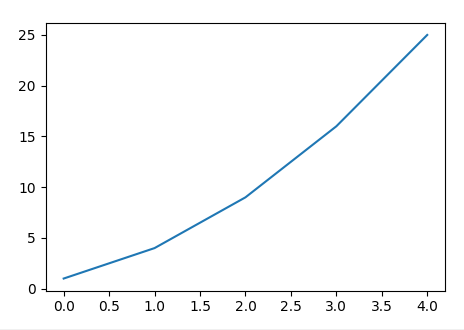为了便于将来更灵活地摆放多个图，推荐下面这种写法：

import matplotlib.pyplot as plt

fig, ax = plt.subplots()

squares = [1, 4, 9, 16, 25]
ax.plot(squares)
fig.show()


## 保存为图片或PDF

fig.savefig(“test-figure.png”, dpi=300)
fig.savefig(“test-figure.pdf”)

## 分别定义x和y

前面的例子实际上省略了x列表，如果x和y都写上，是这样：

import matplotlib.pyplot as plt

fig, ax = plt.subplots()

x=[0, 1, 2, 3, 4]
y=[0, 3, 1, 5, 2]
ax.plot(x, y)
fig.show()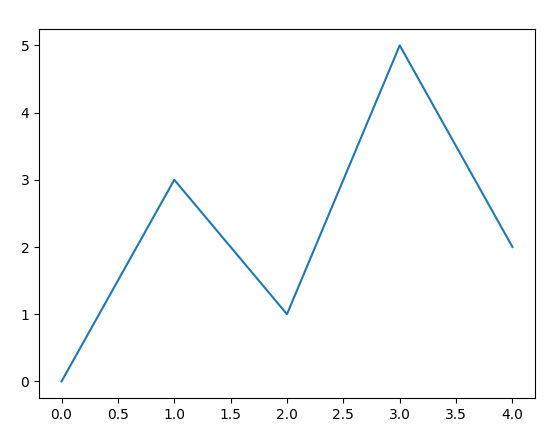## 加上标题、坐标轴标签和网格线

set_title()设置图名。
set_xlabel()和set_ylabel()设置X轴和Y轴的名称。
grid()加网格线。

import matplotlib.pyplot as plt

fig, ax = plt.subplots()

x=[0, 1, 2, 3, 4]
y=[0, 3, 1, 5, 2]
ax.plot(x, y)

ax.set_title('折线图', fontproperties="SimHei")
ax.set_xlabel('X轴', fontproperties="SimSun")
ax.set_ylabel('Y轴', fontproperties="SimSun")
ax.grid(True)

fig.show()## 自定义X轴和Y轴的范围

用set_xlim()和 set_ylim()定义轴的最小刻度和最大刻度值。

import matplotlib.pyplot as plt

fig, ax = plt.subplots()

x=[0, 1, 2, 3, 4]
y=[0, 3, 1, 5, 2]
ax.plot(x, y)

ax.set_title('折线图', fontproperties="SimHei")
ax.set_xlabel('X轴', fontproperties="SimSun")
ax.set_ylabel('Y轴', fontproperties="SimSun")
ax.grid(True)

ax.set_xlim(xmin=-1, xmax=10)
ax.set_ylim(ymin=-1, ymax=6)

fig.show()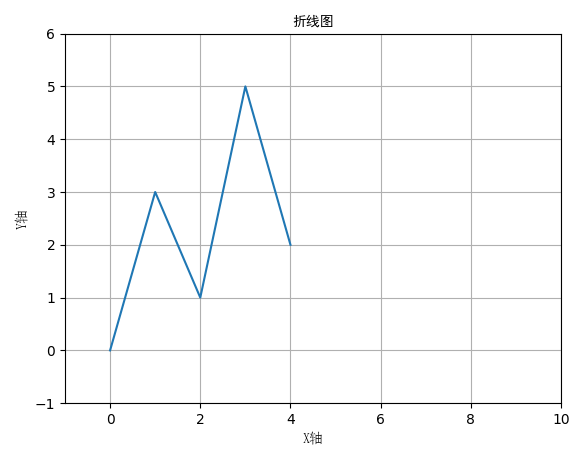## 在数据点上显示标记

plot(x, y, marker=‘o’) 显示圆点标记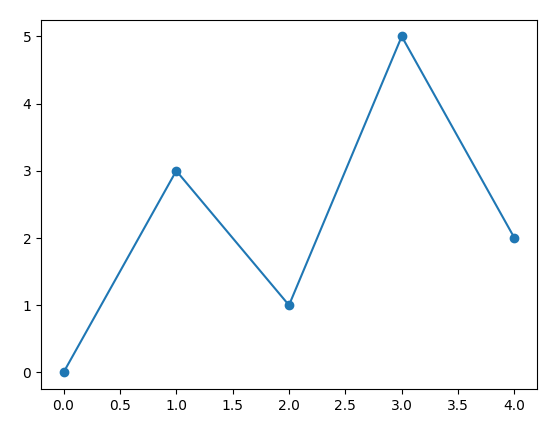还有很多种标记：

• ‘o’ 圆圈 Circle
• ‘s’ 方块标记 Square
• ‘*’ 星形标记 Star
• ‘x’或’X’ 叉
• ‘d’或’D’ 钻石形状标记（菱形） Diamond
• '+‘或’P’ 十字 Plus
• ‘^’ 向上三角
• ‘v’ 下三角
• ‘>’ 左三角
• ‘<’ 右三角
• ‘p’ 五边形 Pentagon
• ‘h’ 或 ‘H’ 六边形 Hexagon
• ‘|’ 竖线
• ‘_’ 横线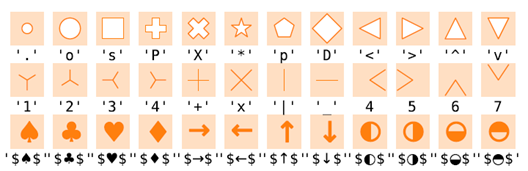## 设置标记的大小，连线的线型、颜色等

plt.plot(x, y, marker=‘o’, markersize=16, linestyle=‘dotted’, color=‘r’)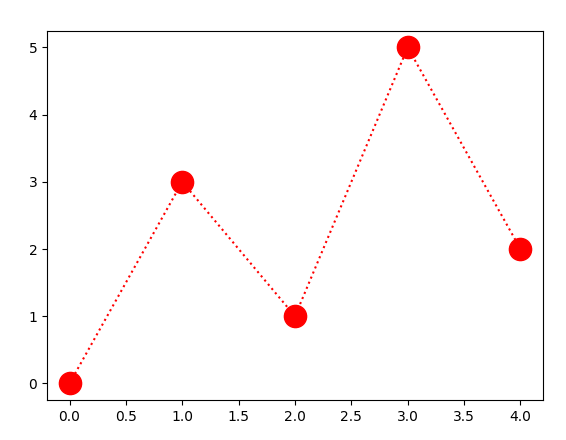线型：
‘-’ 实线 solid
‘:’ 点虚线 dotted
‘–’ 虚线 dashed
‘-.’ 点划线 dashdot

颜色：
可以用一个字母表示的颜色。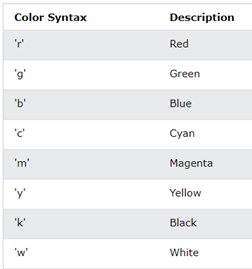还可以这样表示颜色：
（1）一个字母
（2）颜色名称
（3）C0 到 C9
（4）RGB元组，RGBA元组
（5）16进制的0-255的RGB分量，‘#RRGGBB’或‘#RRGGBBAA’
（6）'0.0’到’1.0’的小数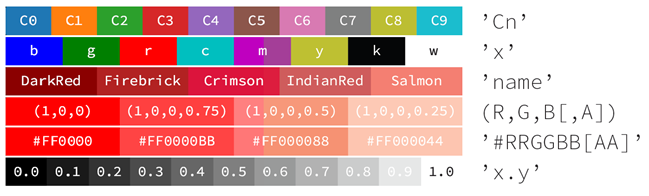# 散点图

plot(x, y, ‘s’) 可以直接画出散点图。
注意与前面折线图的区别：
plot(x, y, marker=‘s’)

import matplotlib.pyplot as plt

fig, ax = plt.subplots()

x=[0, 1, 2, 3, 4]
y=[0, 3, 1, 5, 2]
ax.plot(x, y, 's')
fig.show()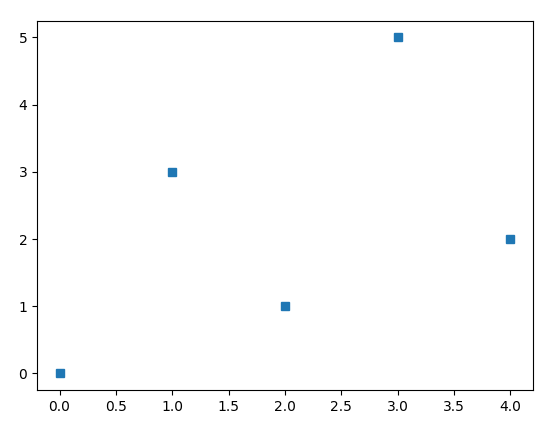更直观的写法是用scatter()

import matplotlib.pyplot as plt

fig, ax = plt.subplots()

x=[0, 1, 2, 3, 4]
y=[0, 3, 1, 5, 2]
ax.scatter(x, y, marker='s')
fig.show()


## 改变字体大小

使用fontsize参数。

import matplotlib.pyplot as plt

fig, ax = plt.subplots()

x=[0, 1, 2, 3, 4]
y=[0, 3, 1, 5, 2]
ax.scatter(x, y, marker='s')

ax.set_title('折线图', fontproperties="SimHei", fontsize=24)
ax.set_xlabel('X轴', fontproperties="SimSun", fontsize=16)
ax.set_ylabel('Y轴', fontproperties="SimSun", fontsize=16)
ax.grid(True)

fig.show()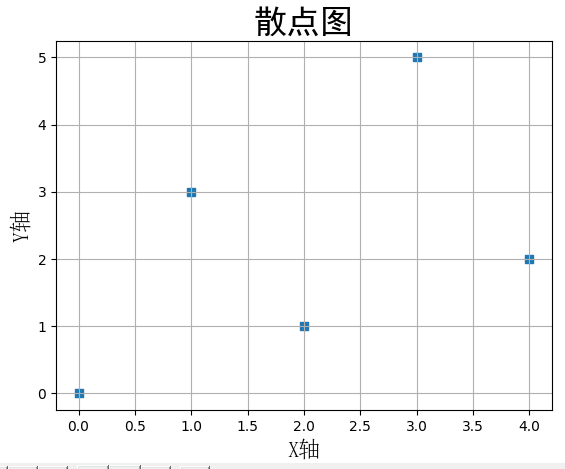## 使用内置样式

有许多内置样式可用。

>>> import matplotlib.pyplot as plt
>>> plt.style.available
['Solarize_Light2', '_classic_test_patch', '_mpl-gallery',
'_mpl-gallery-nogrid', 'bmh', 'classic', 'dark_background',
'fast', 'fivethirtyeight', 'ggplot', 'grayscale', 'seaborn',
'seaborn-bright', 'seaborn-colorblind', 'seaborn-dark',
'seaborn-dark-palette', 'seaborn-darkgrid', 'seaborn-deep',
'seaborn-muted', 'seaborn-notebook', 'seaborn-paper', 'seaborn-pastel',
'seaborn-poster', 'seaborn-talk', 'seaborn-ticks', 'seaborn-white',
'seaborn-whitegrid', 'tableau-colorblind10']


换一种样式：
plt.style.use()

import matplotlib.pyplot as plt

plt.style.use('bmh')

fig, ax = plt.subplots()

x=[0, 1, 2, 3, 4]
y=[0, 3, 1, 5, 2]

ax.scatter(x, y, marker='s')

ax.set_title('折线图', fontproperties="SimHei", fontsize=24)
ax.set_xlabel('X轴', fontproperties="SimSun", fontsize=16)
ax.set_ylabel('Y轴', fontproperties="SimSun", fontsize=16)
ax.grid(True)

fig.show()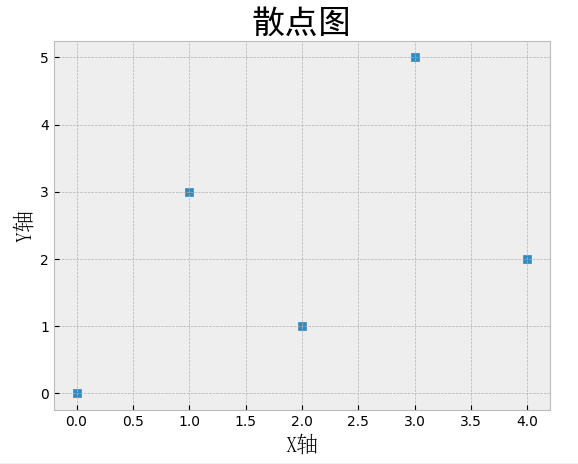## 每个散点可以设置不同的大小和颜色。

import matplotlib.pyplot as plt

plt.style.use('bmh')

fig, ax = plt.subplots()

x=[0, 1, 2, 3, 4]
y=[0, 3, 1, 5, 2]

colors = [1, 2, 3, 1, 1]
size = [100 * c * c for c in colors]
ax.scatter(x, y, s=size, c=colors, alpha=0.5)

fig.show()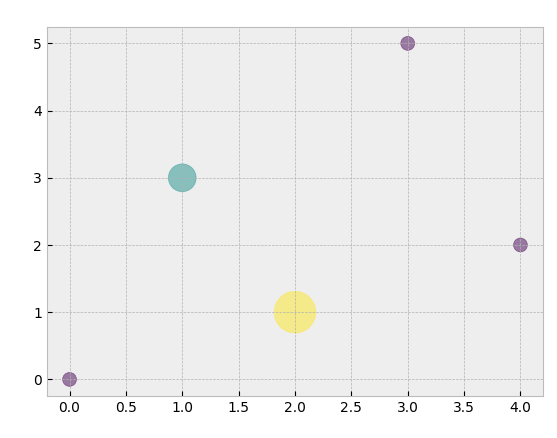展开全文python matplotlib
• echarts 折线散点图 安装相关依赖 npm install echarts-stat <div ref="chart" style="width: 550px; height:310px;"></div> methods: { drawLine() { var data = [ [1, 4862.4], [2, 5294.7], ...echarts
• 绘制散点图 scatter() （图片中各个图表的）方法 参数 参数情况与plot()方法的1-3一致，散点图就不存在线宽的设置而是需要进行点大小的设置。 点的大小（s=某常数）。 点的颜色（c='某颜色的英文'或c=(三个0-1数值...人工智能 神经网络
• Python生成3D图，饼图，合图，散点图折线图，柱状图
• Origin: 双Y轴 | 柱状图 | 折线图 | 垂线散点图的结合绘制 多图层参数设置
• plt.rcParams['font.sans-serif'] = 'SimHei'#设置字体为SimHei显示中文\n", plt.rc('font', size=14)#设置图中字号大小\n", plt.figure(figsize=(6,4)) #绘制折线图，设置颜色和线型\n", plt.plot(x,y1,color = 'r'...python 开发语言 机器学习
• 示例：散点图最常见的散点图之一是x-y散点图。下面的代码会大致告诉你一个matplotlib是如何工作的，你会看到如何一点点建立起一个散点图。我们正在使用点的x和y位置的一些构成数据。运行下面的代码，然后我们将解释...
• 点旁边+号新增一个 https://mirrors.aliyun.com/pypi/simple/  9返回上一层刷新一下就可以搜索想下的jar包了 下载： 1、matplotlib 2、numpy 一、折线图 #导入matplotlib包中的pyplot类,并设置别名为plt import ...pycharm ide python
• 绘制散点图 调整曲线的颜色、粗细 调整图标标签 绘制简单折线图 折线图的绘制，需要用到matplotlib.pyplot库，利用其中的plot()方法实现折线图的绘制。 import matplotlib.pyplot as plt input_values=[1,2,3,4,5] ...python 折线图 散点图
• matlab散点图折线图When you were learning algebra back in high school, you might not have realized that one day you would need to create a scatter plot to demonstrate real-world results. 当您在高中学习...
• 想要的效果 ...散点图显示错位 option = { xAxis: { type: 'category' data: [1,2] }, yAxis: {}, series: [{ symbolSize: 20, data: [ [10.0, 8.04], [8.07, 6.95] ], type: 'scatter' }echarts
• 1.折线图 折线图通常用来表示数据随时间或有序类别变化的趋势。 '''1.简单示例''' import matplotlib.pyplot as plt data = [1,2,3,4,5,4,2,6,9,2] # 数据 plt.plot(data) plt.show() '''2.绘制多条曲线、曲线...matplotlib 散点图 折线图
• 默认情况下是绘制散点图，也可以绘制线性图，具体绘制什么图形是通过kind参数来决定的。实际上以下两个函数就是relplot的特例： • 散点类型：scatterplot -> relplot(kind=“scatter”) • 线性类型：lineplot -...python
• ## python画散点图、折线图

万次阅读 多人点赞 2020-12-10 22:54:24
Python-画图（散点图scatter、保存savefig）及颜色大全 import numpy as np import matplotlib.pyplot as plt plt.rcParams['font.sans-serif']=['SimHei'] plt.rcParams['axes.unicode_minus'] = False #...python 开发语言 后端 django
• 目录 1、散点图绘制 2、折线图绘制 1、散点图绘制 代码1：sin(x)点绘制 from pyecharts.charts import Scatter import pyecharts.options as opts import numpy as np x = np.linspace(0, 10, 50) # 从0开始，到10...python 散点图...